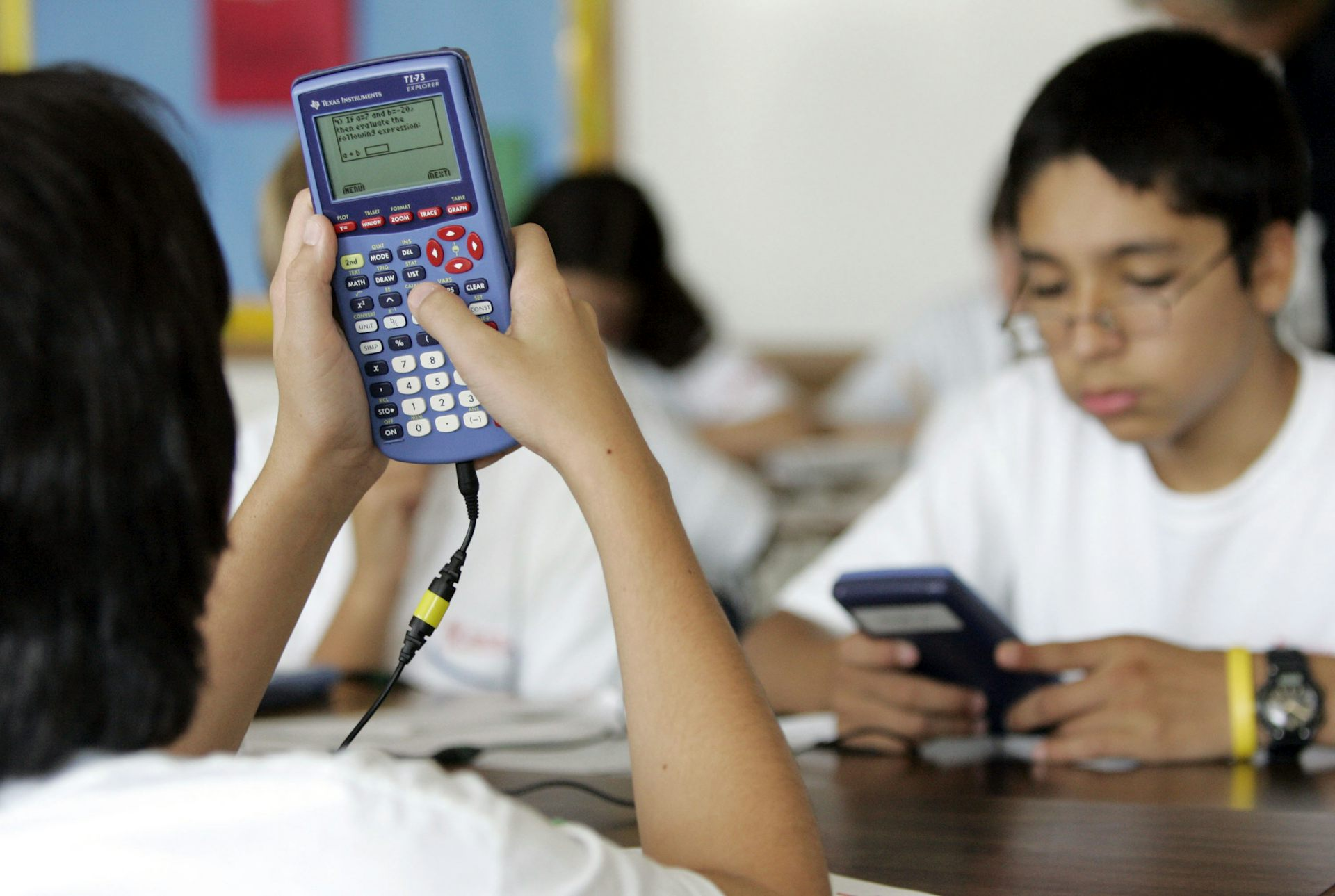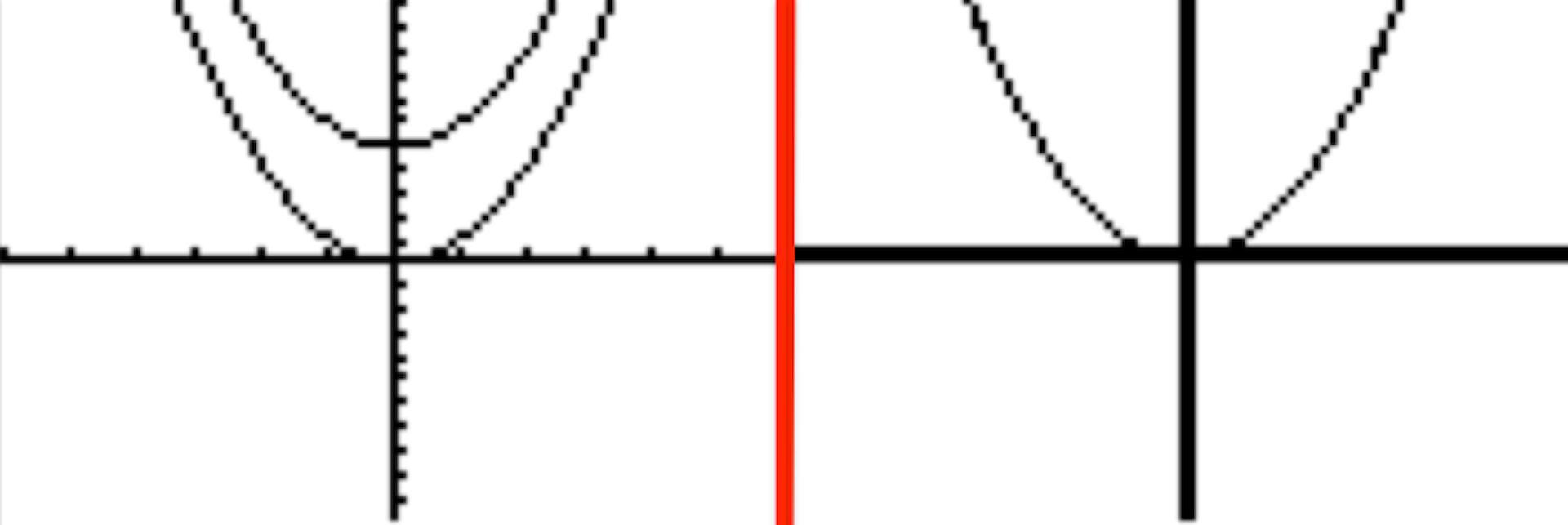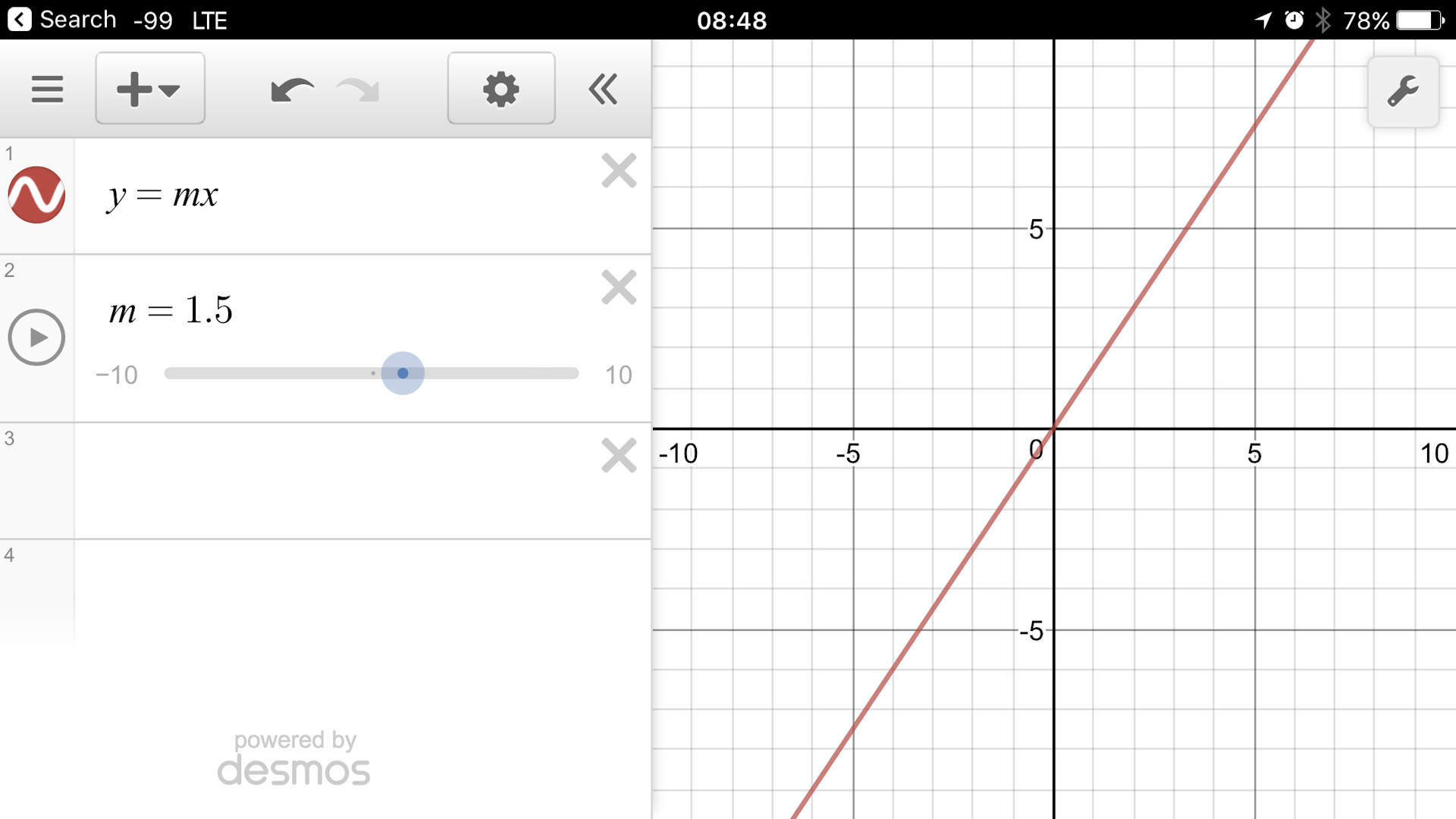Graphing calculators – like the ones used in this seventh grade Dallas classroom – have become ubiquitous in U.S. education. AP Photo/Tony Gutierrez

The first handheld graphing calculator, the Casio fx-7000G, appeared in 1985.

Since then, graphing calculators have become a common – and controversial – tool for learning mathematics. These devices can do all of the calculations of a scientific calculator, plus graph equations, make function tables and solve equations. Many have the ability to do statistical analysis and even some calculus.

Advocates claim that the calculators provide students access to more powerful mathematics. Critics worry that they might hurt students’ fluency in basic math and standard algorithms.

Today, some teachers are replacing expensive graphing calculators with free apps that can do more. But even after decades of use, graphing technology of any sort in the classroom still sparks debate.

As mathematics educators, we think the graphing calculator transformed American classrooms for the better. Whether teachers continue to use these tools or ditch them in favor of new ones, graphing technology will likely always have a place in secondary math education.

# Understanding math

Math educators often talk about two kinds of understanding.

“Instrumental understanding” comes from mastering a procedure or memorizing a fact, without really understanding the mathematics behind it. It’s knowing how, but not why. The saying “It is not ours to wonder why, just invert and multiply!” – which is sometimes used to teach division of fractions – captures this sort of understanding.

In contrast, “relational understanding” is a kind of connected, conceptual understanding. People with a relational understanding don’t just know how to invert and multiply, they know why such a procedure results in the quotient of two fractions.

Advocates for graphing calculators in school saw promise in the tool’s ability to help students develop relational understanding. While the calculator takes care of the “how,” students can focus on “why.”

The impact is quite clear in the Advanced Placement (AP) calculus program, which started to require graphing calculators in their courses and on their exams in 1995. Prior to 1995, AP calculus exam questions probed almost solely for the students’ ability to use rules to find derivatives and integrals of functions. After 1995, there was a marked shift away from this instrumental understanding and toward questions that probed for relational understanding.

As exams evolved, so too did teaching philosophies. The AP program required teachers to use graphing calculators in their courses. This was not just so students would learn how to use the calculator. Rather, the focus of instruction shifted so that students could learn mathematics through the graphing calculator.

For example, by using the graphing and zoom features of the graphing calculator, students could compare and contrast the local and global behavior of functions such as y = x² and y = x² + 2. By zooming in, students can see that in any local area, the graphs are clearly different. By zooming out, students can see that globally, the graphs are basically identical. Through exploration such as this, students gained relational understanding of infinite limits.In the local area (-6 < x < 6) shown at left, the graphs of y = x² + 5 and y = x² are clearly different. But zooming out (at right), the graphs of the two functions are basically identical. Frederick Peck, CC BY

# Does the tech help or hurt?

Still, some teachers wondered whether this shift in instruction in AP calculus and across the K-12 curriculum would have a negative effect on students’ instrumental understanding. After all, if a machine is doing the calculating for you, why bother to learn it?

After more than three decades of research, the findings are clear. Graphing calculators have a positive effect on students’ relational understanding and a slight positive effect on their instrumental understanding.

Another review, conducted by respected researchers in math education (but funded by a calculator company), came to a similar conclusion.

In other words, students who use graphing calculators in school know at least as many basic facts and are at least as good at doing standard algorithms as students who do not use graphing calculators. However, students who use graphing calculators have a deeper understanding of the “whys” behind those algorithms.

Of course, there are many individual studies that show negligible or even negative effects of graphing calculators. But overall, when the technology is paired with appropriate instructional techniques, the result is more and better mathematics learning.

# The rise of graph apps

Today, online and app-based technology, such as Desmos and GeoGebra, aim to replace the role of stand-alone graphing calculators in school.

As with graphing calculators, for these new technologies to have a positive effect on student learning, teachers have to adapt their instruction, changing what they teach and how they teach it.

For example, technology can help students connect graphical representations with algebraic equations. We recently observed seventh grade students in Missoula, Montana doing that using Desmos. Students graphed three different linear equations, each with a different coefficient for the x-term. Based on this exploration, students made conjectures about the role of the coefficient, and used the app to test their conjectures – for example, by using a “slider” to dynamically vary the coefficient. The flexibility of the technology also encouraged students to pose and explore their own questions.On the Desmos app, students can test their questions by dynamically varying the coefficient using a slider. David Erickson

Even as these cloud- and app-based tools provide powerful technology for free on smartphones and other personal devices, the expensive graphing calculator (which typically costs between US\$80 and \$150) continues to be a stalwart in K-12 math classes, with year-over-year units sales increasing in 2015 and 2016.

There has recently been much online hand-wringing over this state of affairs, with commentators aghast at the continued dominance of graphing calculators when compared to inexpensive or free apps. The debate even provoked a response from the president of Texas Instruments, the dominant graphing calculator company in the U.S.

We agree with many of the points in favor of the new apps. But there is no doubt that, when properly used, graphing technology – whether on a calculator, computer screen, tablet or smartphone – is a powerful tool for helping students learn mathematics.

### Want to write?

Write an article and join a growing community of more than 95,100 academics and researchers from 3,098 institutions.